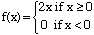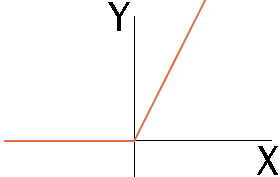Name: Jenny Who is asking: Student Level: Secondary Question: How do you figure out a piecewise function by hand? e.x.        ( 2x (if x is not equal to 0) f(x)=<        ( 0 (if x is not equal to 0) Hi Jenny, I am not sure that I have the inequalities that way you have but I think that your function is something like:This is a way to describe a function that in part of its domain looks like f(x) = 0, that is the x-axis, and in part of its domain looks like f(x) = 2x, the straight line through the origin with slope 2. The division point is at the zero on the x-axis. If x is less than 0 then the function is described by f(x) = 0 and if x is greater than or equal to 0 the function is described by f(x) = 2x. Its graph is:Cheers, Harley Go to Math Central